2017/07/25 07:32

1、图，

2、宽度最优搜索,

3、Dijkstra 算法，

4、贪心算法，

5、A*搜索算法,

6、B*搜索算法,

# 1、游戏中的人物是如何寻路的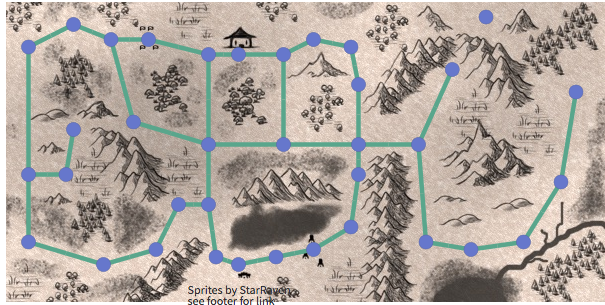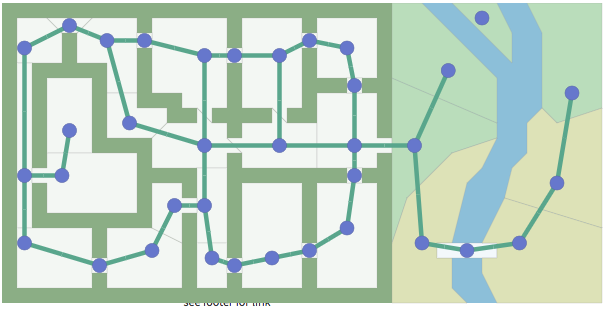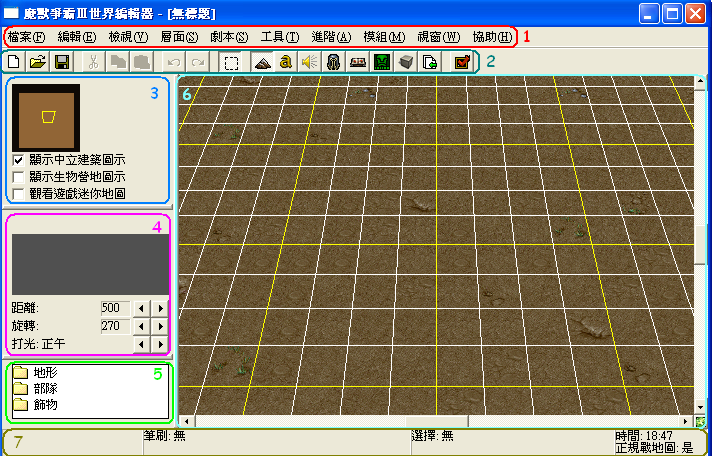# 2、图

### 图的基本定义: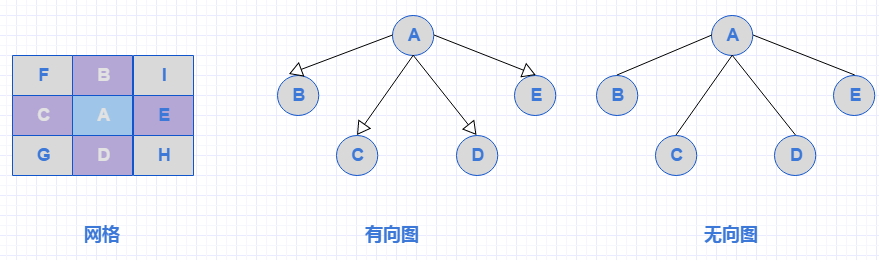# 3、搜索算法

## 广度优先搜索

BFS是一种盲目搜寻法，目的是系统地展开并检查中的所有节点，以找寻结果。换句话说，它并不考虑结果的可能位址，彻底地搜索整张图，直到找到结果为止它的步骤如下:

- 首先将根节点放入队列中。

- 从队列中取出第一个节点，并检验它是否为目标。

- 如果找到目标，则结束搜寻并回传结果。

- 否则将它所有尚未检验过的直接子节点（邻节点）加入队列中。

- 若队列为空，表示整张图都检查过了——亦即图中没有欲搜寻的目标。结束搜寻并回传“找不到目标”。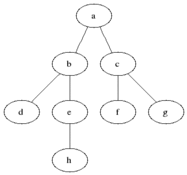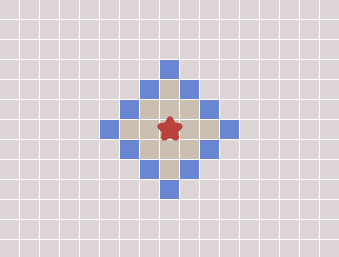var frontier = new Array();

frontier.put(start);

var visited = new Array();

visited[start] = true;

while(frontier.length>0){

current = frontier.get();

//查找周围顶点

for(next in graph.neighbors(current)){

var notInVisited = visited.indexOf(next)==-1;

//没有访问过

if(notInVisited) {

frontier.put(next);

visited[next] = true;

}

}
}

### 缺陷:

1、效率底下， 时间复杂度是:T(n) = O(n^2)

2、每个顶点之间没有权值，无法定义优先级，不能找到最优路线。比如遇到水域需要绕过行走，在宽度算法里面无法涉及。

# 4、Dijkstra 算法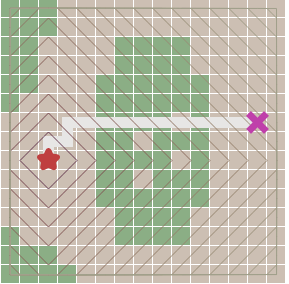### 解决痛点:

Dijkstra 算法基于宽度优先算法进行改进，把当前看起来最短的边加入最短路径树中 ，利用贪心算法计算并最终能够产生最优结果的算法。具体步骤如下:

1、每个顶点都包含一个预估值cost(起点到当前顶点的距离)，每条边都有权值v ，初始时，只有起始顶点的预估值cost为0，其他顶点的预估值d都为无穷大 ∞。

2、查找cost值最小的顶点A，放入path队列

3、循环A的直接子顶点，获取子顶点当前cost值命名为current_cost，并计算新路径new_cost，new_cost=父节点A的cost+v(父节点到当前节点的边权值),如果new_cost<current_cost,当前顶点的cost=new_cost

4、重复2，3直至没有顶点可以访问.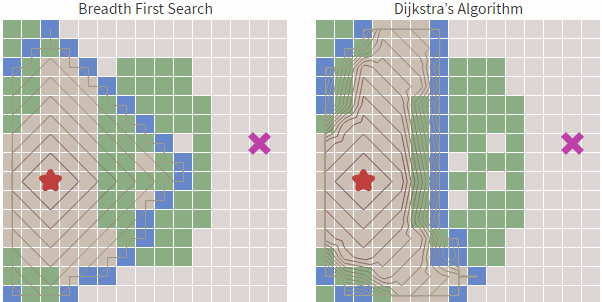var frontier = new PriorityQueue();

frontier.put(start);

path = new Array();

//每个顶点路径消耗

cost_so_far = new Array();

path[start] = 0;

cost_so_far[start] = 0

while(frontier.length>0){

current = frontier.get();

if current == goal:

break

//查找周围节点

for(next in graph.neighbors(current)){

var notInVisited = visited.indexOf(next)==-1;

var new_cost = cost_so_far[current] + graph.cost(current, next);

//没有访问过或者路径更近

if(notInVisited ||  new_cost < cost_so_far[next]) {

cost_so_far[next] = new_cost;

priority = new_cost;

frontier.put(next, priority);

path[next] = current;

}

}

}

### 缺点:

1、运行时间复杂度是:T(n) = O(V^2)，其中V为顶点个数。效率上并不高

2、目标查找不具有方向性

# 5、贪婪最佳优先搜索

### 解决痛点: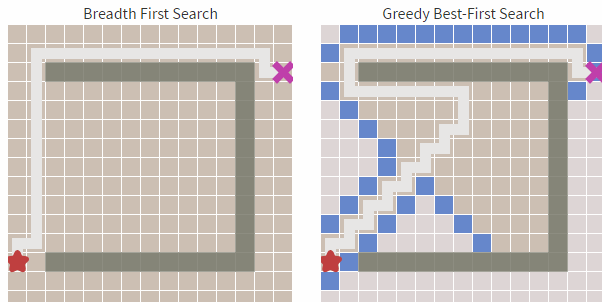frontier = new PriorityQueue();

frontier.put(start, 0)

came_from = new Array();

came_from[start] = 0;

while(frontier.length>0){

current = frontier.get()

if current == goal:

break

for(next in graph.neighbors(current)){

var notInVisited = visited.indexOf(next)==-1;

//没有访问过

if(notInVisited ) {

//离目标的距离 ，距离越近优先级越高

priority = heuristic(goal, next);

frontier.put(next, priority);

came_from[next] = current;

}

}

}

function heuristic(a, b){

//离目标的距离

return abs(a.x - b.x) + abs(a.y - b.y)

}

1.路径不是最短路径，只能是较优

# 6、A*算法

A*算法的优先队列排序方式基于F值:

F=cost(顶点到起始顶点的距离 )+heuristic(顶点到目标顶点的距离 )

var frontier = new PriorityQueue();

frontier.put(start);

path = new Array();

cost_so_far = new Array();

path[start] = 0;

cost_so_far[start] = 0

while(frontier.length>0){

current = frontier.get()

if current == goal:

break

for(next in graph.neighbors(current)){

var notInVisited = visited.indexOf(next)==-1;

var new_cost = cost_so_far[current] + graph.cost(current, next);

//没有访问过而且路径更近

if(notInVisited ||  new_cost < cost_so_far[next]) {

cost_so_far[next] = new_cost

//队列优先级= new_cost(顶点到起始顶点的距离 )+heuristic(顶点到目标顶点的距离 )

priority = new_cost + heuristic(goal, next)

frontier.put(next, priority)

path[next] = current

}

}

}

function heuristic(a, b){

//离目标的距离

return abs(a.x - b.x) + abs(a.y - b.y)

}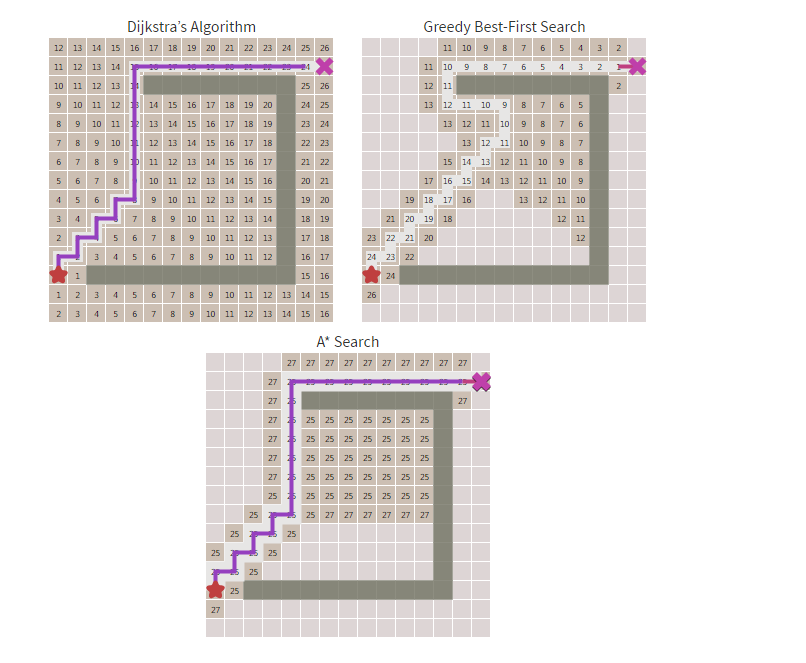# 7、B*算法

----------------------------------------end-----------------------------------12 评论
150 收藏
11